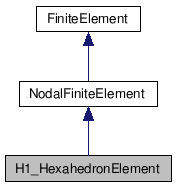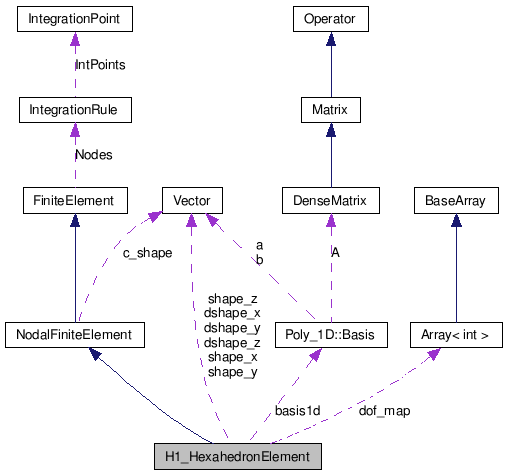MFEM v2.0
H1_HexahedronElement Class Reference

`#include <fe.hpp>`

Inheritance diagram for H1_HexahedronElement:[legend]
Collaboration diagram for H1_HexahedronElement:[legend]

List of all members.

## Public Member Functions

H1_HexahedronElement (const int p)
virtual void CalcShape (const IntegrationPoint &ip, Vector &shape) const
virtual void CalcDShape (const IntegrationPoint &ip, DenseMatrix &dshape) const

## Private Attributes

Poly_1D::Basisbasis1d
Vector shape_x
Vector shape_y
Vector shape_z
Vector dshape_x
Vector dshape_y
Vector dshape_z
Array< int > dof_map

## Detailed Description

Definition at line 1261 of file fe.hpp.

## Constructor & Destructor Documentation

 H1_HexahedronElement::H1_HexahedronElement ( const int p )

Definition at line 6121 of file fe.cpp.

## Member Function Documentation

 void H1_HexahedronElement::CalcDShape ( const IntegrationPoint & ip, DenseMatrix & dshape ) const` [virtual]`

pure virtual function which evaluates the values of all partial derivatives of all shape functions at a given point ip and stores them in the matrix dshape (Dof x Dim) so that each row contains the derivatives of one shape function

Implements FiniteElement.

Definition at line 6228 of file fe.cpp.

 void H1_HexahedronElement::CalcShape ( const IntegrationPoint & ip, Vector & shape ) const` [virtual]`

pure virtual function which evaluates the values of all shape functions at a given point ip and stores them in the vector shape of dimension Dof

Implements FiniteElement.

Definition at line 6209 of file fe.cpp.

## Member Data Documentation

 Poly_1D::Basis& H1_HexahedronElement::basis1d` [private]`

Definition at line 1264 of file fe.hpp.

Referenced by CalcDShape(), and CalcShape().

 Array H1_HexahedronElement::dof_map` [private]`

Definition at line 1268 of file fe.hpp.

Referenced by CalcDShape(), CalcShape(), and H1_HexahedronElement().

 Vector H1_HexahedronElement::dshape_x` [mutable, private]`

Definition at line 1266 of file fe.hpp.

Referenced by CalcDShape(), and H1_HexahedronElement().

 Vector H1_HexahedronElement::dshape_y` [mutable, private]`

Definition at line 1266 of file fe.hpp.

Referenced by CalcDShape(), and H1_HexahedronElement().

 Vector H1_HexahedronElement::dshape_z` [mutable, private]`

Definition at line 1266 of file fe.hpp.

Referenced by CalcDShape(), and H1_HexahedronElement().

 Vector H1_HexahedronElement::shape_x` [mutable, private]`

Definition at line 1266 of file fe.hpp.

Referenced by CalcDShape(), CalcShape(), and H1_HexahedronElement().

 Vector H1_HexahedronElement::shape_y` [mutable, private]`

Definition at line 1266 of file fe.hpp.

Referenced by CalcDShape(), CalcShape(), and H1_HexahedronElement().

 Vector H1_HexahedronElement::shape_z` [mutable, private]`

Definition at line 1266 of file fe.hpp.

Referenced by CalcDShape(), CalcShape(), and H1_HexahedronElement().

The documentation for this class was generated from the following files: